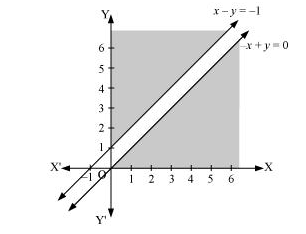# MaximiseQuestion:

Maximise $Z=x+y$, subject to $x-y \leq-1,-x+y \leq 0, x, y \geq 0$.

Solution:

The region determined by the constraints, $x-y \leq-1,-x+y \leq 0, x, y \geq 0$, is as follows.There is no feasible region and thus, Z has no maximum value.